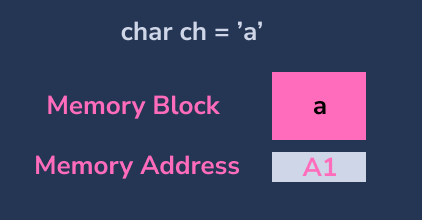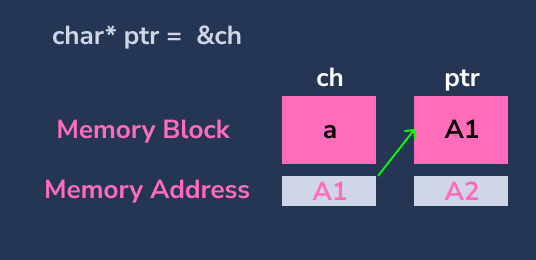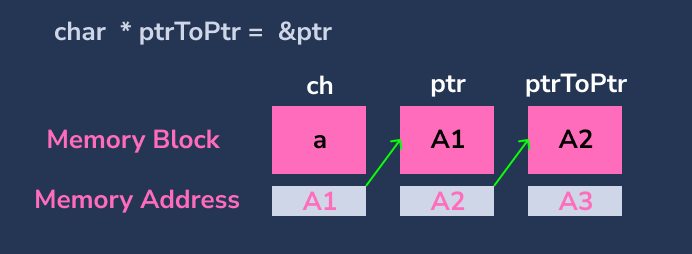# ** in C

This tutorial teaches how to use the Pointer to Pointer(Double pointer or `**` ) to store the address of another pointer variable.

## Memory Allocation for Variable in C

On creating a variable, there will be some specific block of memory allocated to that variable for storing the value. For example, we have created a `char` variable `ch` and value `a`. Internally, one byte of memory will be allocated to the variable `ch`.## C Pointer

In C programming, a pointer is a variable that stores the address of another variable. To access the value present in that address, we use `*`.``````#include <stdio.h>

int main()
{
char ch = 'a'; // create a variable
char *ptr = &ch; // create a pointer to store the address of ch

printf("Value of ch: %c\n", ch);  // prints 'a'

printf("\nValue of ptr: %p\n", ptr);  // prints the address of a
printf("*ptr(value of ch): %c\n", *ptr);  // Prints Content of the value of the ptr
}
``````

Output:

``````Address of ch: 0x7ffc2aa264ef
Value of ch: a

Value of ptr: 0x7ffc2aa264ef
*ptr(value of ch): a
``````

In the above code,

• Created a `char` variable `ch` and assigned the character `a` as a value.
• Created a `char` pointer `ptr` and stored the address of the variable `ch`.
• Printed the address and value of `ch`.
• Printed the value of the `ptr`, the value of `ptr` will be the address of `ch`
• Printed the value of `ch` by using `*ptr`. The value of `ptr` is the address of the variable `ch`, in that address, the value `'a'` is present, so it will be printed.

## Pointer to Pointer (`**`) in C

To store the address of a variable, we use a pointer. Similarly, to store the address of a pointer, we need to use `**` (pointer to pointer). `**` denotes a pointer that stores the address of another pointer.

To print the value present in the pointer to pointer variable, we need to use `**`.``````#include <stdio.h>

int main()
{
char ch = 'a'; // create a variable
char *ptr = &ch; // create a pointer to store the address of ch
char **ptrToPtr = &ptr; // create a pointer to store the address of ch

printf("Value of ch: %c\n", ch);  // prints 'a'

printf("\nValue of ptr: %p\n", ptr);  // prints the address of ch

printf("\nValue of ptrToPtr: %p\n", ptrToPtr);  // prints the address of ptr
printf("**ptrToPtr(Value of ch): %c\n", **ptrToPtr);  // prints ch
}
``````

Output:

``````Address of ch: 0x7fffb48f95b7
Value of ch: a

Value of ptr: 0x7fffb48f95b7

Value of ptrToPtr: 0x7fffb48f95b8
**ptrToPtr(Value of ch): a
``````

In the above code,

• Created a `char` variable `ch` and assigned the character `a` as a value to it.
• Created a `char` pointer `ptr` and stored the address of the variable `ch`.
• Created a `char` pointer to pointer `ptrToPtr` and stored the address of the variable `ptr`.
• The ptr will have the address of the variable `ch` as value, and the `ptrToPtr` will have the address of pointer `ptr` as value.
• When we dereference the `ptrToPtr` like `*ptrToPtr` we get address of variable `ch`
• When we dereference the `ptrToPtr` like `**ptrToPtr` we get value of variable `ch`

### Point to Remember

In order to store the address of `ptrToPtr` we need to create

``````char ***ptrToPtrToPtr = &ptrToPtr;
printf("***ptrToPtrToPtr : %c\n", ***ptrToPtrToPtr);  // 'a'
``````
Write for us
DelftStack articles are written by software geeks like you. If you also would like to contribute to DelftStack by writing paid articles, you can check the write for us page.

## Related Article - C Pointer

• Function Pointer in C
• Use Pointer Ampersand Notation in C
• Fix Free Invalid Pointer Error in C